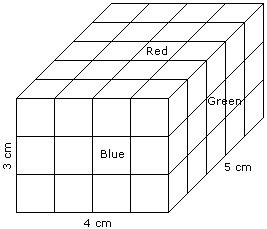# Verbal Reasoning - Cube and Cuboid

Exercise : Cube and Cuboid - Cube and Cuboid 3
Directions to Solve

The following questions are based on the information given below:

1. A cuboid shaped wooden block has 4 cm length, 3 cm breadth and 5 cm height.
2. Two sides measuring 5 cm x 4 cm are coloured in red.
3. Two faces measuring 4 cm x 3 cm are coloured in blue.
4. Two faces measuring 5 cm x 3 cm are coloured in green.
5. Now the block is divided into small cubes of side 1 cm each.

1.
How many small cubes will have will have three faces coloured ?
14
8
10
12
Explanation:Such cubes are related to the corners of the cuboid and in the cuboid there are 8 corners.

Hence, the required number of small cubes is 8.

2.
How many small cubes will have only one face coloured ?
12
28
22
16
Explanation:2 from the front + 2 from the back + 3 from the left + 3 from the right + 6 from the top + 6 from the bottom = 22

3.
How many small cubes will have no faces coloured ?
None
2
4
6
Explanation:Required number of small cubes = (5 - 2) x (4 - 2) x (3 - 2)

= 3 x 2 x 1

= 6

4.
How many small cubes will have two faces coloured with red and green colours ?
12
8
16
20